Java Games: Flashcards, matching, concentration, and word search.# Math Review MS

AB
Multiply by 10add a zero or move decimal one place to the right
Multiply by 100add two zeroes or move decimal two places to the right
Divide by 10remove one zero or move decimal one place to the left
Divide by 100remove two zeroes or move decimal two places to the left
expanded form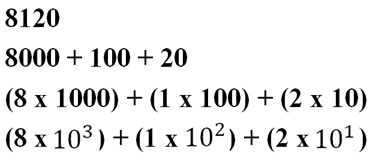scientific notationexpanded form with decimals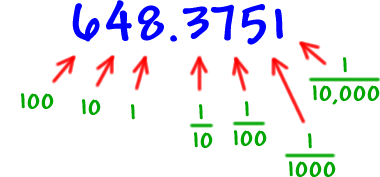order of operations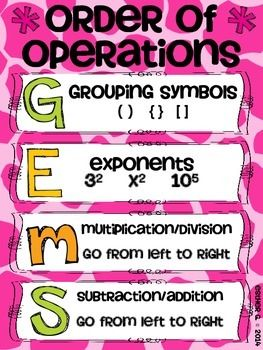base ten systemordered pairs/coordinate plane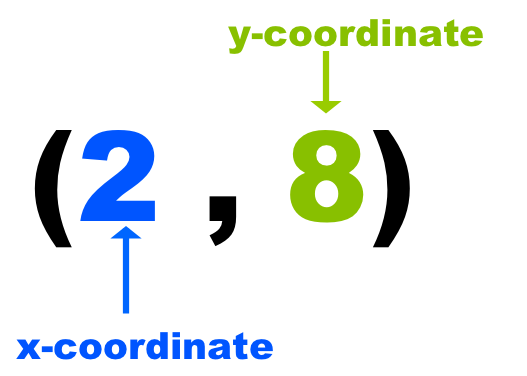exponents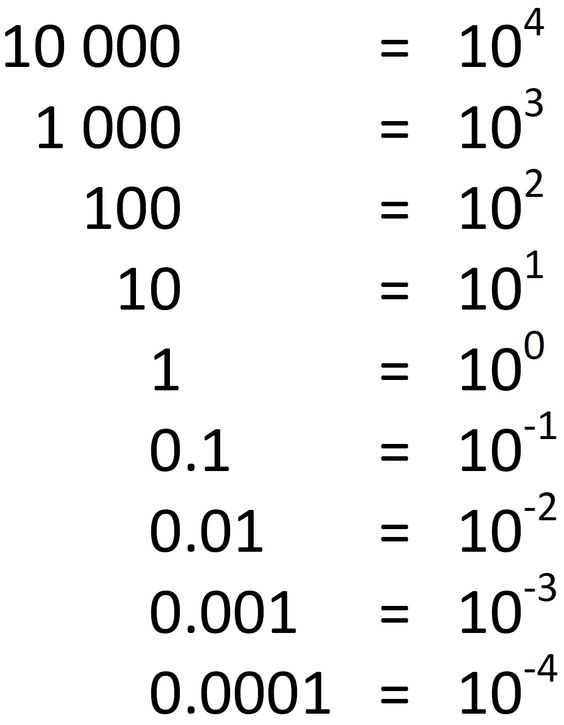tenths, hundredths, thousandths place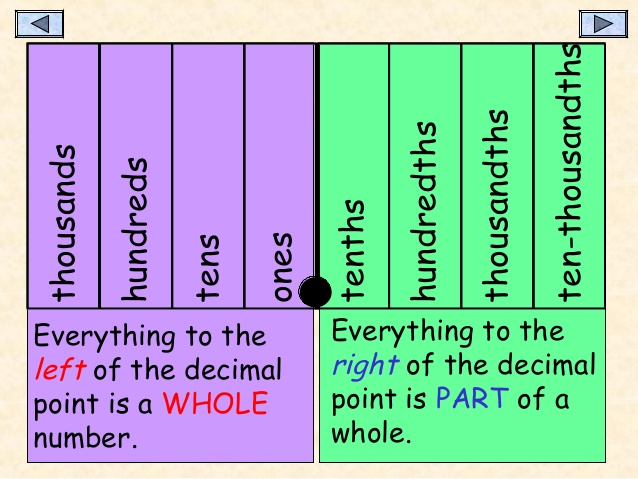arrays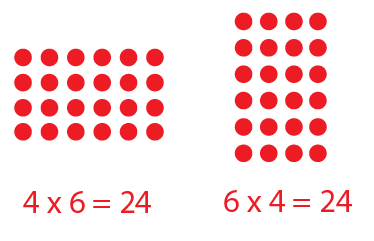dividend, divisor, quotient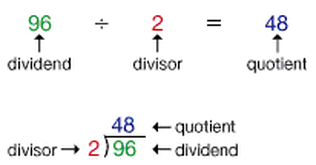add, subtract, multiply, and divide by decimals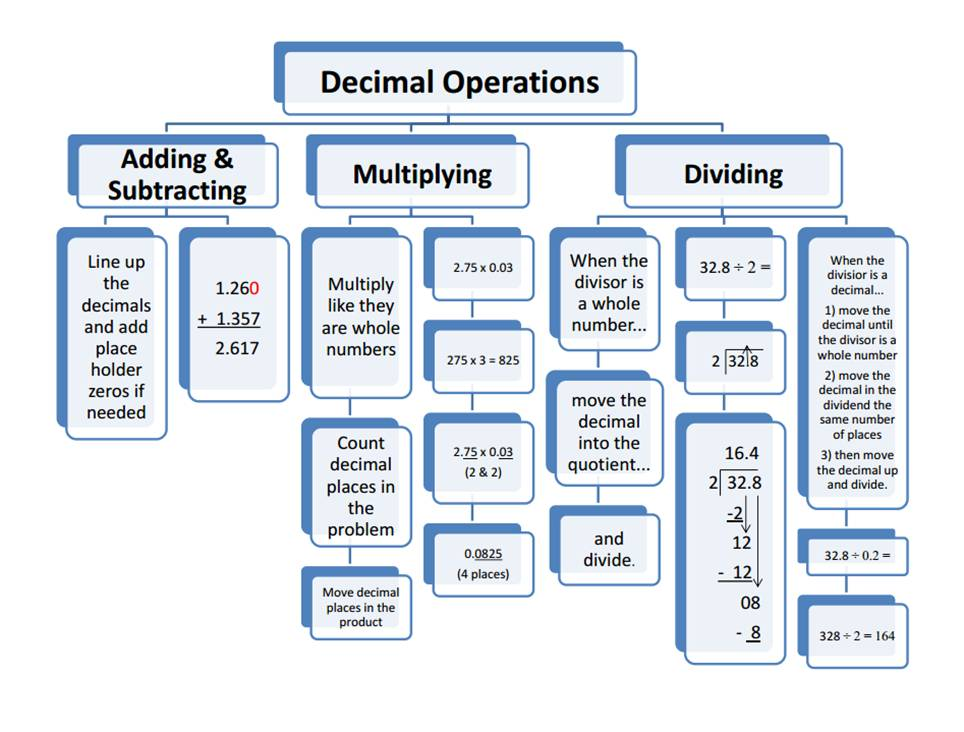equivalent fractions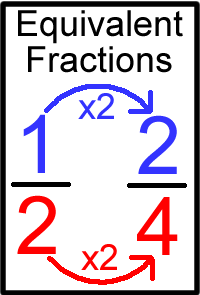common denominators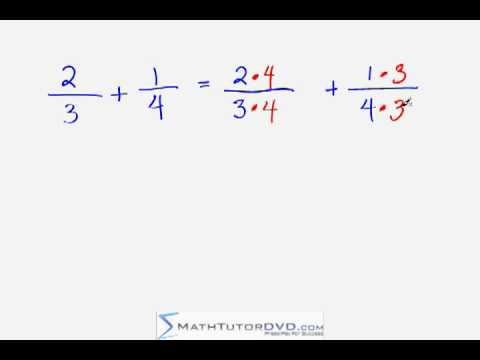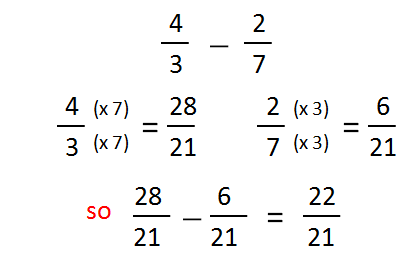benchmark fractionsis it closer to 0, 1/2, or 1 whole
fractions as division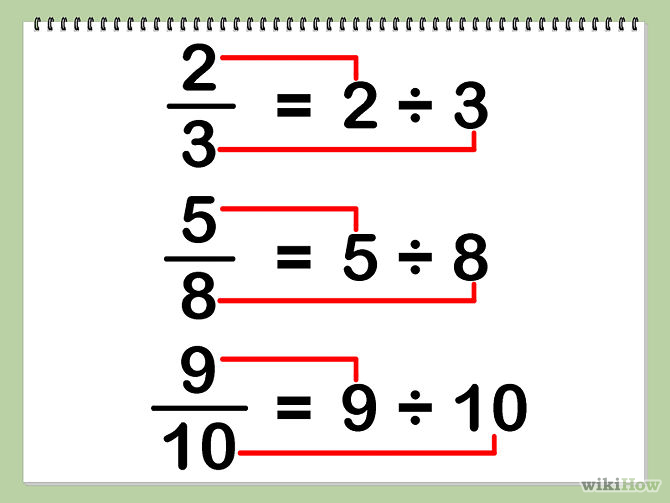whole number times fraction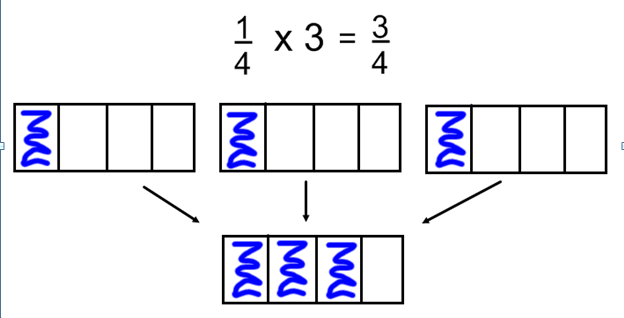arealength times width,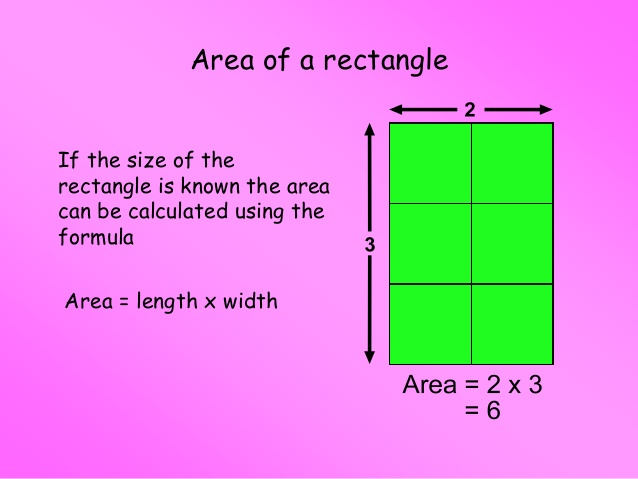kilogram to gram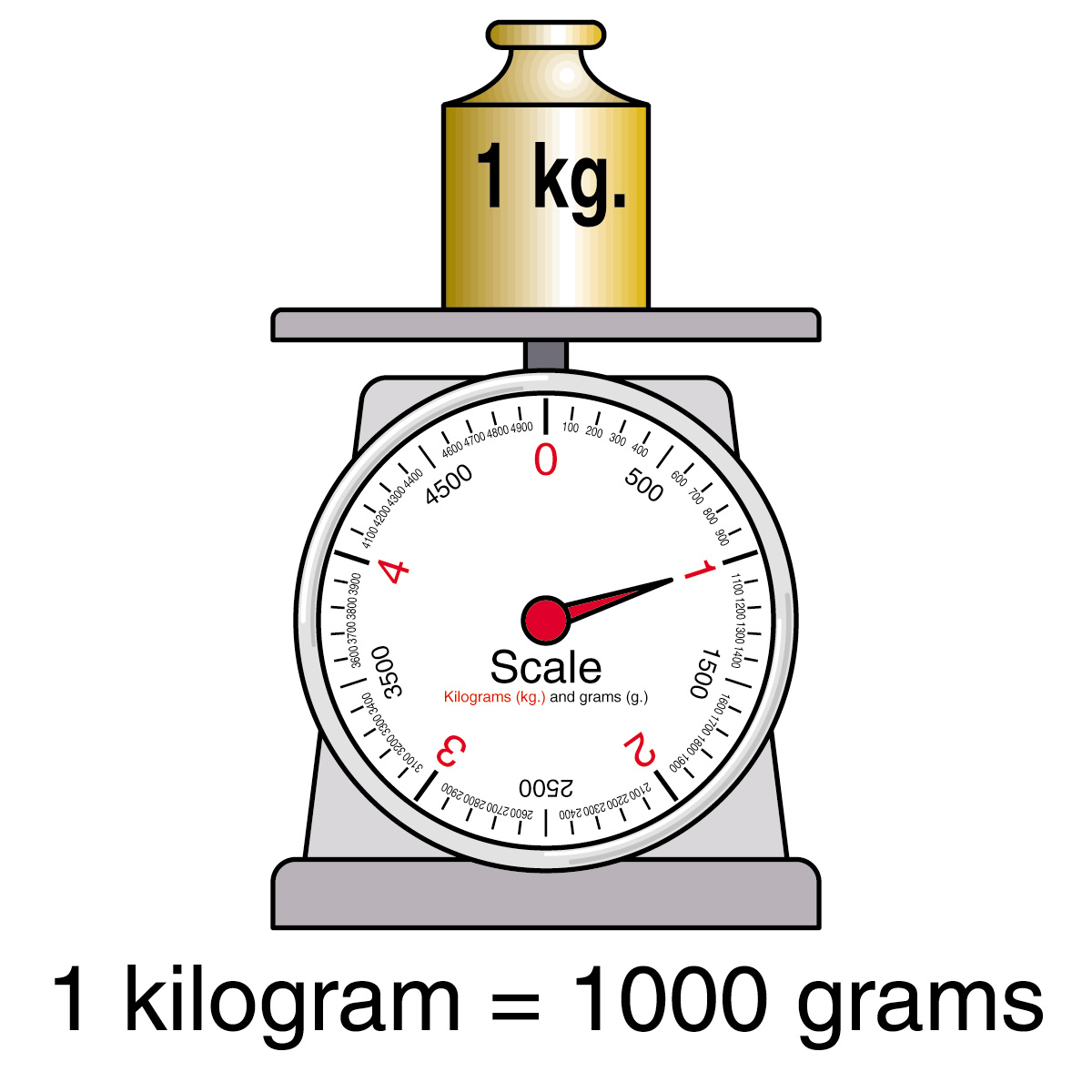kilogram to pounds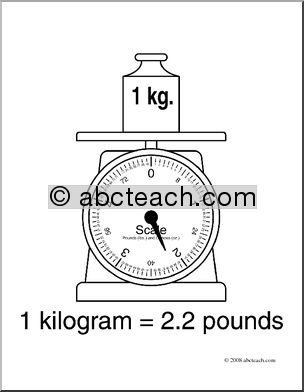gram to milligramunits of massKilograms, grams, milligrams - how much matter is in something or how much space it takes up
units of weightPounds, tons, ounces-Measure the pull of gravity on something,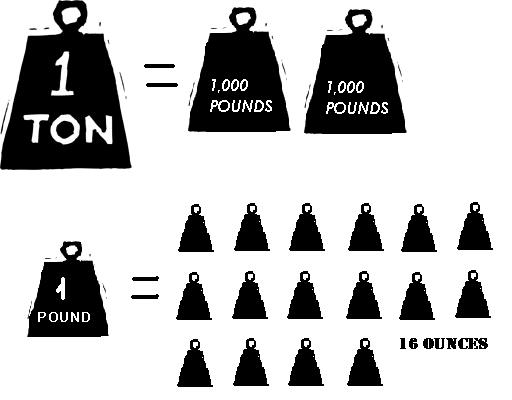units of length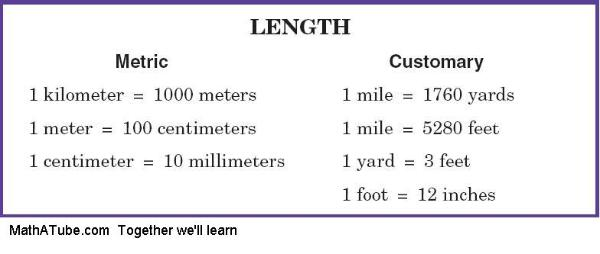line plots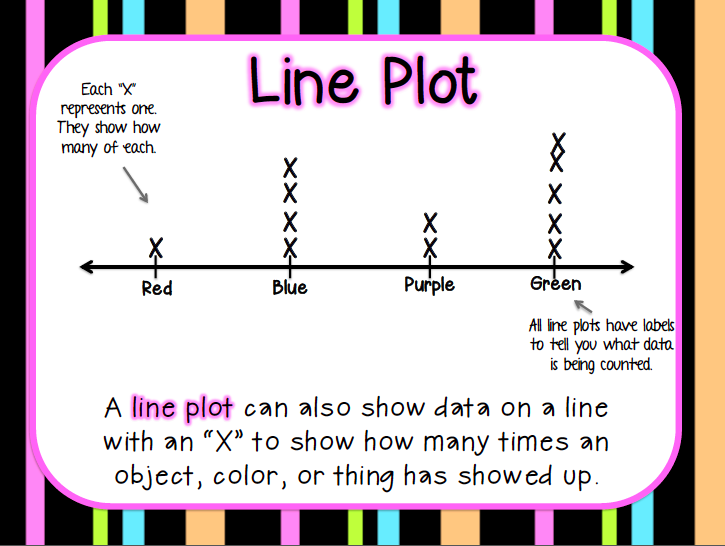polygon characteristics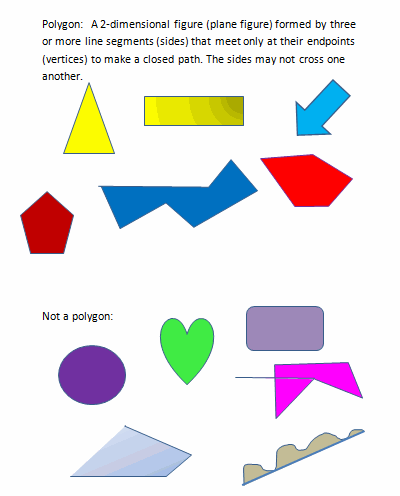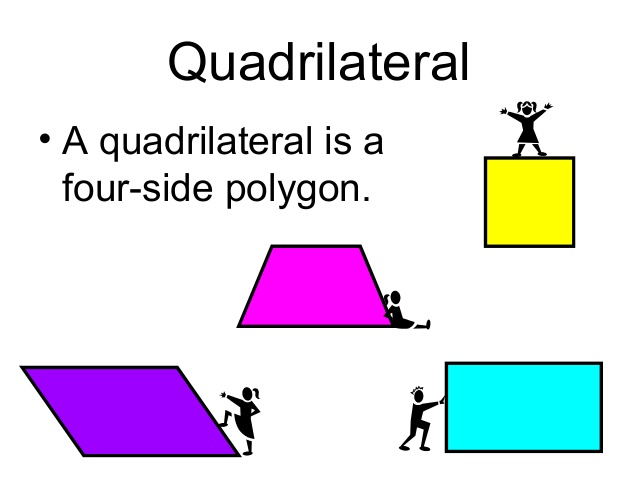types of trianglestypes of polygons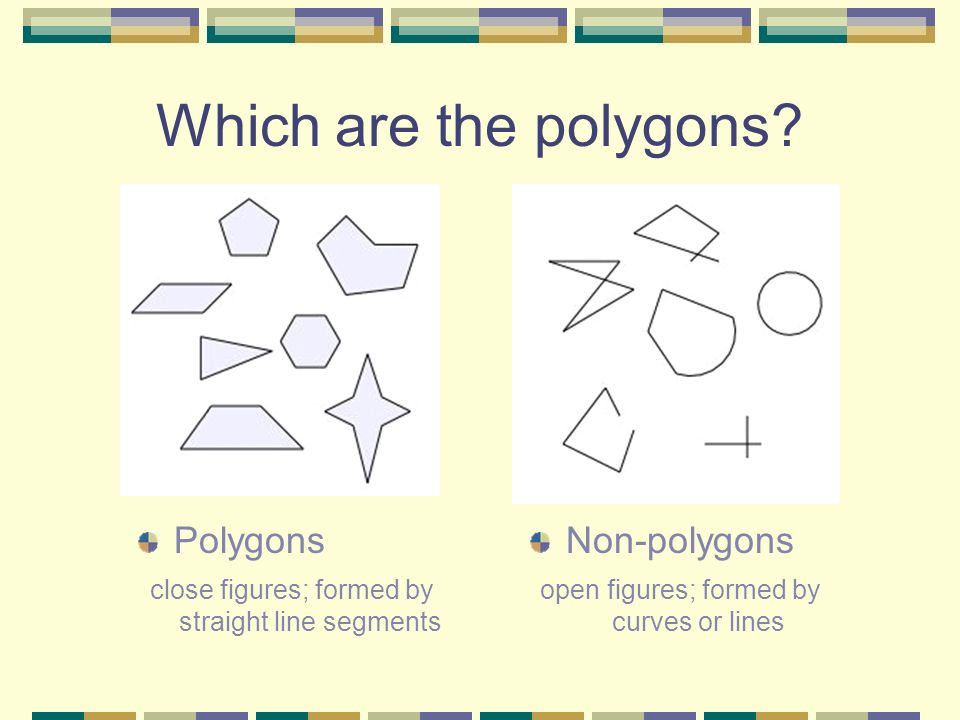regular and irregular polygonsregular polygons are equilateral (all sides the same) and equiangular (all angles the same),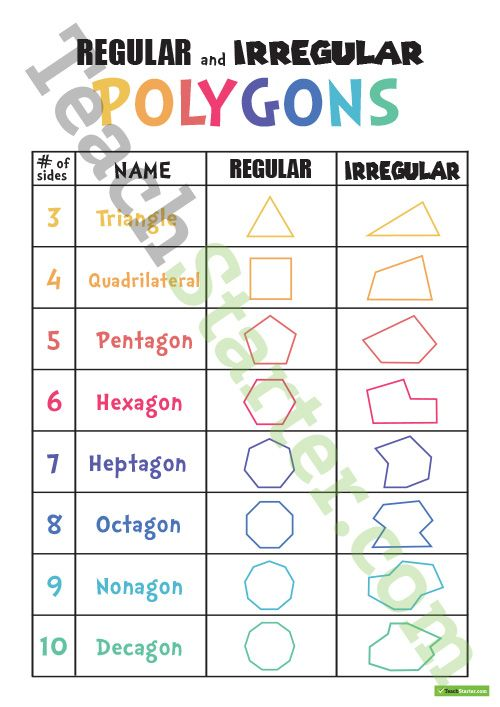coordinate planes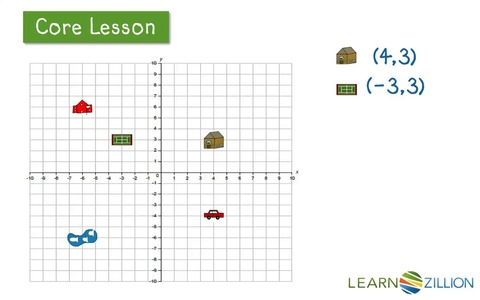labeling a coordinate planeThe origin is always ZERO,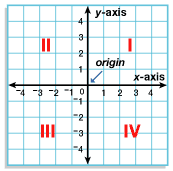symmetrycreating a mirror image of something
lines of symmetry in regular polygonssame as number of sides,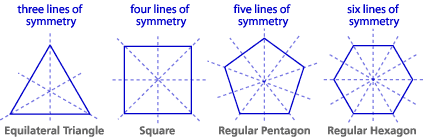volume calculationlength times width times height,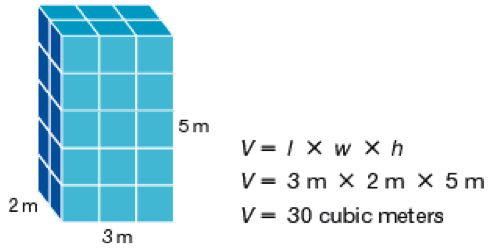cubic unitcount these to determine the volume of a 3D object- can be measured in inches, centimeters, feet, yard, etc.,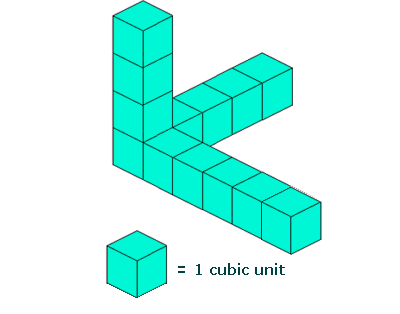Volume of two prisms added togetherfind the volume of each individual prism and then add together,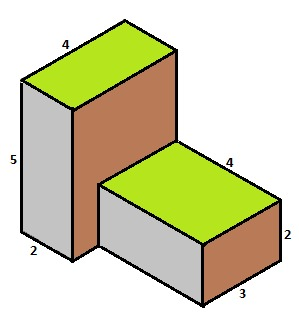squarefour side that are the same and four right angles
area of a quadrilateral in degrees360 degrees
area of triangle in degrees180 degrees
parallel linesthey will never cross or meet,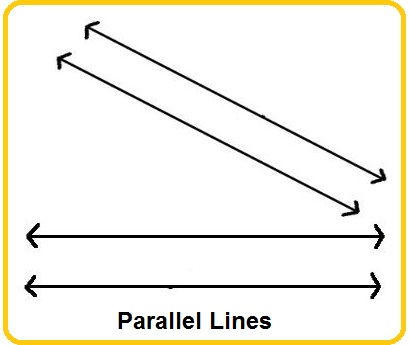perpendicular linestwo lines that make a right angle,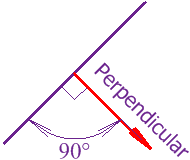mixed number to improper fractionimproper to mixed number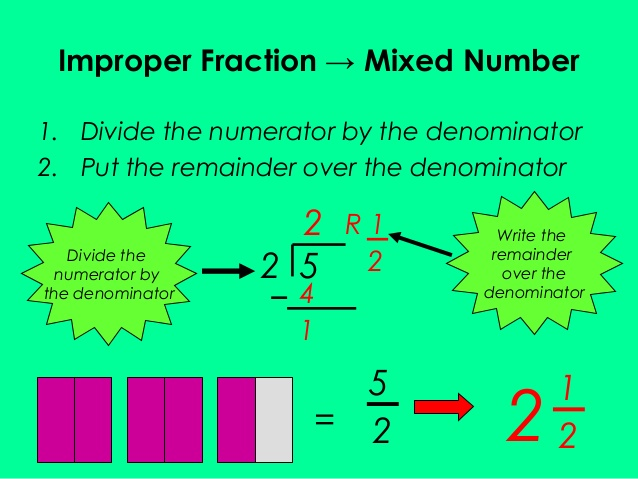Whole number divided by fraction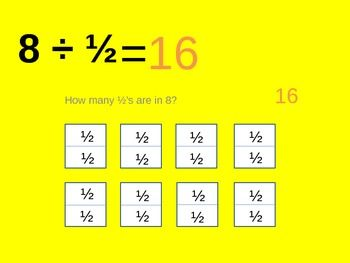Fraction divided by whole number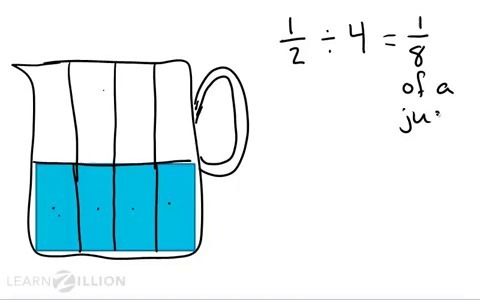ton2000 pounds
gallon4 quarts
quart2 pints
pint2 cups
meter100 centimeters; 1000 millimeters
mile5280 feet
foot12 inches
liter1000 milliliters
centi1/100th
milli1/1000th
kilo1000
deci1/10th
.77/10ths or .70
.055/100ths or five cents
divisible by 2even,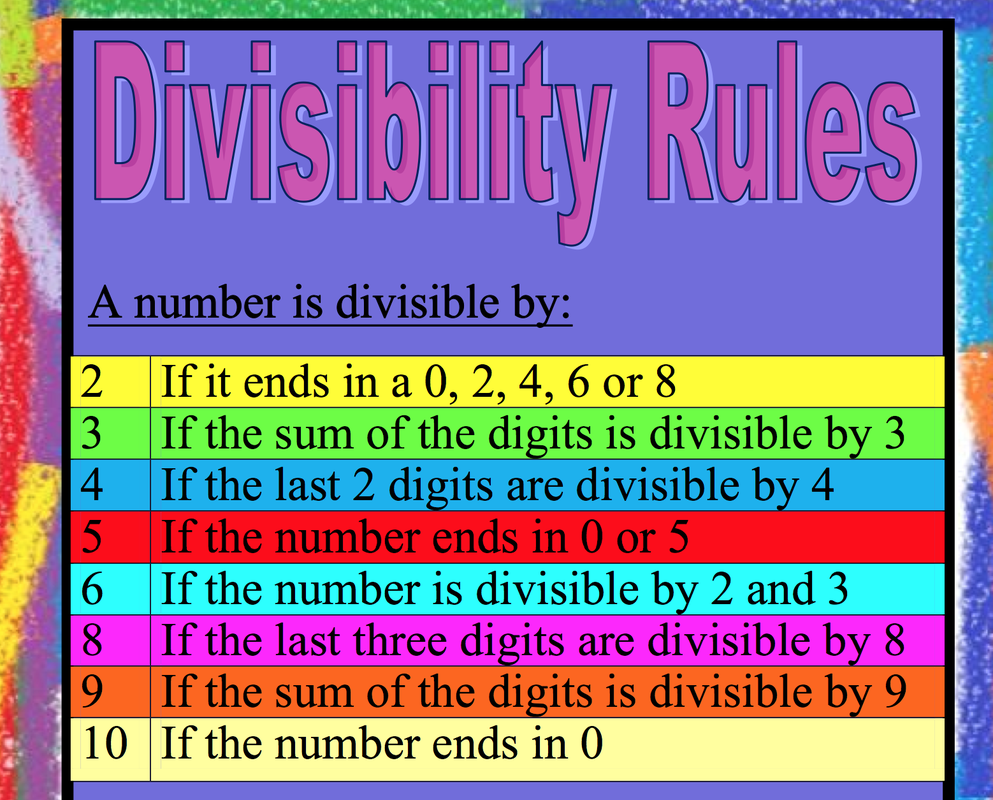divisible by 50 or 5 in one's place,divisible by 100 in one's place,divisible by 3add numbers together and is multiple of 3,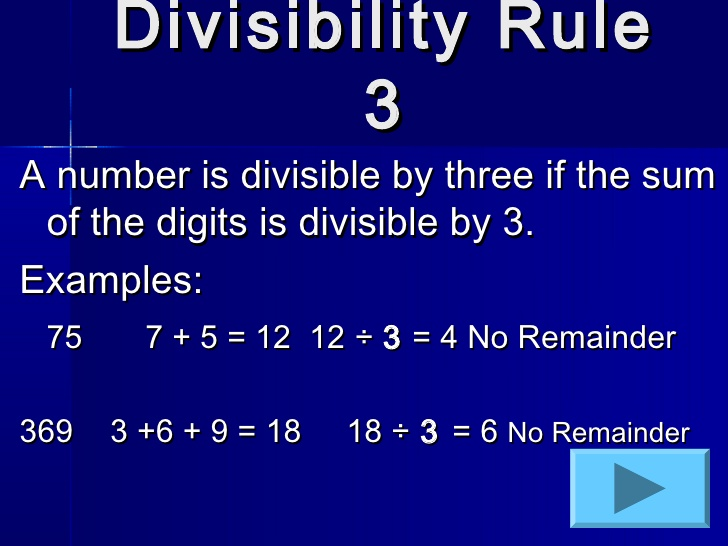multiplesthe number times 1, 2, 3, 4, 5....,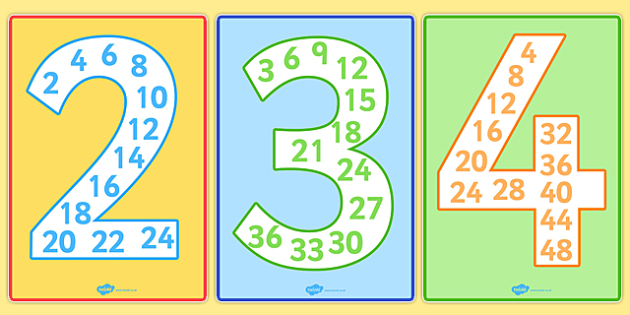fraction and decimal equivalence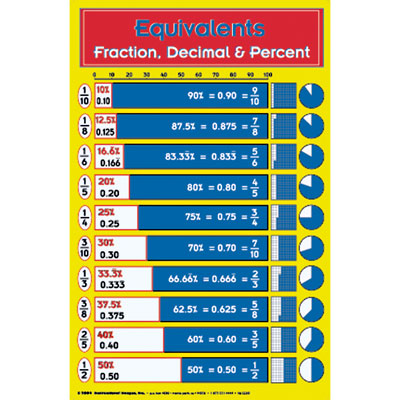This activity was created by a Quia Web subscriber. Learn more about QuiaCreate your own activities